UnboundEd Mathematics Guide

# Number & Operations in Base Ten: Unbound A Guide to Grade 5 Mathematics Standards

?
What's in a Content Guide and how do I use it?
Get answers to all your Content Guide questions, including what's in each part and how they can be used in your role at your school
View FAQs

5.NBT.A | Understand the place value system.

5.NBT.B | Perform operations with multi-digit whole numbers and with decimals to hundredths.

5.MD.A | Convert like measurement units within a given measurement system.

Welcome to the UnboundEd Mathematics Guide series! These guides are designed to explain what new, high standards for mathematics say about what students should learn in each grade, and what they mean for curriculum and instruction. This guide, the first for Grade 5, includes three parts. The first part gives a “tour” of the standards in the Number & Operations in Base Ten domain using freely available online resources that you can use or adapt for your class. The second part shows how Number & Operations in Base Ten relates to other concepts in Grade 5. And the third part explains some important progressions of learning that lead to (and extend from) these standards in Grade 5. Throughout all of our guides, we include a large number of sample math problems. We strongly suggest tackling these problems yourself to help best understand the methods and strategies we’re covering, and the potential challenges your students might face.

# Part 1: What do the standards say?

In Grade 5, the Number & Operations in Base Ten (NBT) domain is composed of two clusters; each cluster has associated standards and is part of the major work of Grade 5, as indicated by the green square.1 The Common Core State Standards for Mathematics (CCSSM) are organized into major, additional, and supporting clusters in the Focus by Grade Level documents from Student Achievement Partners. The Common Core State Standards for Mathematics (CCSSM) are organized into major, additional, and supporting clusters in the Focus by Grade Level documents from Student Achievement Partners. The Common Core State Standards for Mathematics (CCSSM) are organized into major, additional, and supporting clusters in the Focus by Grade Level documents from Student Achievement Partners. A large majority of time should be spent teaching the major work of the grade. The other cluster discussed in this guide comes from the Measurement & Data (MD) domain. This is a supporting cluster (blue square). Supporting clusters enhance the focus and coherence of the major clusters. The standards in this MD cluster can help students deepen their understanding of the standards in the NBT clusters. We will talk about these standards in detail in Part 2.

It’s important to note that the clusters, and the standards within the clusters, are not necessarily sequenced in the order in which they have to be taught. (Standards are only a set of expectations of what students should know and be able to do by the end of each year; they don’t prescribe an exact sequence or curriculum.) So planning your instruction sequence carefully can ensure your students continue to build on previous understandings. As we go, think about the connections you see between standards, and how you can use these connections to help students build on their previous understandings.

Beginning the year with study of the NBT standards is just one of several options in Grade 5, but it has its advantages. For example, starting off working with the four operations serves as a nice “refresher” for students, as they have been learning about addition and subtraction since Kindergarten and multiplication and division since Grade 3. It also connects closely with work on place value in Grade 4. Finally, students will be using the four operations all year in a variety of contexts, so starting off with some experiences with them will support later learning.

Throughout the guide, we’ll look at examples of tasks and lessons that focus on students’ abilities to look for and make use of structure (MP.7), as students understand and explain place value and use place value to perform operations.

The first cluster in the Number & Operations in Base Ten domain has four standards. Let’s begin by reading these standards, and then we’ll think through what they mean and how they look in practice.

5.NBT.A | Understand the place value system

 5.NBT.A.1 Recognize that in a multi-digit number, a digit in one place represents 10 times as much as it represents in the place to its right and 1/10 of what it represents in the place to its left. 5.NBT.A.2 Explain patterns in the number of zeros of the product when multiplying a number by powers of 10, and explain patterns in the placement of the decimal point when a decimal is multiplied or divided by a power of 10. Use whole-number exponents to denote powers of 10. 5.NBT.A.3 Read, write and compare decimals to thousandths. 5.NBT.A.3.A Read and write decimals to thousandths using base-ten numerals, number names, and expanded form, e.g., 347.392 = 3 × 100 + 4 × 10 + 7 × 1 + 3 × (1/10) + 9 × (1/100) + 2 × (1/1000). 5.NBT.A.3.B Compare two decimals to thousandths based on meanings of the digits in each place, using >, =, and < symbols to record the results of comparisons. 5.NBT.A.4 Use place value understanding to round decimals to any place.

There’s a lot in there about place value, decimals and more! In cluster NBT.A, it’s important to note the emphasis on conceptual understanding. A lot of the language looks familiar (“reading,” “writing,” “comparing,” “rounding,” “digits,” “decimals”) but the cluster heading (“Understand the place value system”) and some of the key verbs (“recognize,” “explain”) help us see that getting students to a deep understanding of place value is our central goal. Not only is this challenging for us as teachers, it’s also different from the way that many of us learned place value and operations in base ten. We’ll revisit this idea as we discuss some examples.

## Place value

Place value understanding in Grade 5 is the culmination of a great deal of prior work with place value, fractions and decimals. We will elaborate on this prior work in Part 3 of this guide; however, in this case it’s important to know what understandings students cultivated in Grade 4, so that the Grade 5 expectations are clear and so that instruction can coherently draw on prior learning in these three areas:

• Place Value: Students learn that for multi-digit whole numbers, a digit in one place represents 10 times as much as it represents in the place to its right (e.g., In the number 3,910, the value of the digit in the hundreds place is 10 times as much the value of the digit in the tens place in the number 391, as 900 ÷ 90 = 10). (4.NBT.A.1)
• Fractions: Students multiply fractions by whole numbers (e.g., 3 × (1/10) = 3/10 and 9 × (1/100) = 9/100) (4.NF.B.4)
• Decimals: Students use decimal notation for fractions with denominators of 10 and 100 (e.g. 3/10 = 0.3 and 9/100 = 0.09). (4.NF.C.6)

Combining these leads students to the critical understanding in Grade 5 that:

• For multi-digit numbers (now including decimals), a digit in one place represents 10 times as much as it represents in the place to its right, and represents 1/10 times as much as it represents in the place to its left. (5.NBT.A.1)

For example, let’s look closely at 4.2 and 42. The value of the digit 2 in 42 is 10 times the value of the digit 2 in 4.2 (i.e., 0.2 x 10 = 2), and the value of the digit 2 in 4.2 is 1/10 the value of the digit 2 in 42 (i.e., 2 x (1/10) = 0.2).

The task below is meant to help students consider the relationship between digits in different places, namely that one place is 10 times the place to its right or 1/10 times the place to its left.

#### Which Number Is It?

Netta drew a picture on graph paper: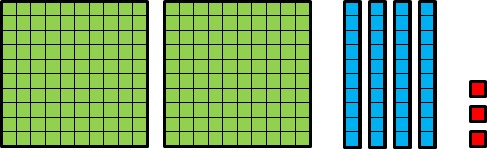She said,

In my picture, a big square represents 1. Since ten rectangles make a big square, a rectangle represents 0.1. Since 100 little squares make a big square, a little square represents 0.01. So this picture represents 2.43.

1. Is Netta Correct?

Manny said,

I drew the same picture, but in my picture, a little square represents 1, so this picture represents 243.

1. Name some other numbers that this picture could represent. For each of these numbers, what does a little square represent? What does a rectangle represent? What does a big square represent? Explain.

1. Draw a picture to represent 0.047.

Let’s also note a few characteristics of this task that work to build conceptual understanding:

• Students are asked to respond to another students’ reasoning. (Part a: “Is Netta correct?”)
• Students are asked to explain their thinking. (Part b: “What does a big square represent? Explain.”)
• Students are asked to interpret visual models in different ways (Part a: interpreting Netta’s model; and Part b: Name some other numbers this picture could represent) and draw visual models. (Part c: Draw a picture to represent 0.047)

Justifying, explaining and using models are some common structures that serve to deepen students’ understanding of concepts and should be a big part of what students do with place value in Grade 5.

## Reading, writing and comparing decimals

In addition to understanding place value, students in Grade 5 will read and write decimals to the thousandths and represent them with base-ten numerals, number names, and expanded form. (5.NBT.A.3.A) They’ll also compare decimals to the thousandths. (5.NBT.A.3.B) The following task asks students to compare decimals to the thousandths and use explanations and representations to support their reasoning. Note again the inclusion of the directives to explain and create drawings; in this case, the drawings help reinforce the relationship between each decimal place.

#### Drawing Pictures to Illustrate Decimal Comparisons

1. Which is greater, 0.01 or 0.001? Explain. Draw a picture to illustrate your explanation.

1. Which is greater, 0.03 or 0.007? Explain. Draw a picture to illustrate your explanation.

1. Which is greater, 0.025 or 0.052? Explain. Draw a picture to illustrate your explanation.

1. Which is greater, 0.13 or 0.031? Explain. Draw a picture to illustrate your explanation.

1. Which is greater, 0.203 or 0.21? Explain. Draw a picture to illustrate your explanation.

Solution

1. 0.01>0.001 because 0.01 is 10 times bigger than 0.001.

In the picture, a small square represents 0.001 and ten of those small squares represents 0.01.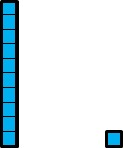1. 0.03 > 0.007 because it takes 10 thousandths to make 1 hundredth, so 7 thousandths is smaller than 1 hundredth which is smaller than 3 hundredths.

In the picture, a small square represents 0.001 and ten of those small squares represents 0.01.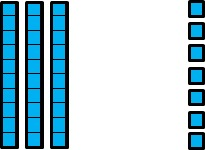1. 0.025<0.052 because there are more hundredths in 0.052 than in 0.025, and hundredths are 10 times bigger than thousandths.

In the picture, a small square represents 0.001 and ten of those small squares represents 0.01. The pictures of the two numbers are stacked to make comparing them easier.1. 0.13>0.031 because it takes 10 hundredths to make 1 tenth, so 0.031 is less than 1 tenth and 0.13 is greater than 1 tenth.

In the picture, a small square represents 0.001, ten of those small squares represents 0.01, and 100 of those small squares represents 0.1. The pictures of the two numbers are stacked to make comparing them easier.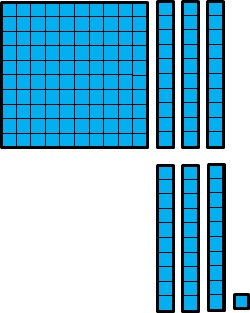1. Lastly, 0.21>0.203. They both have 2 tenths, but 0.21 has 1 hundredth, thereby making it greater than 0.203 which has 0 hundredths. In the picture, a small square represents 0.001, ten of those small squares represents 0.01, and 100 of those small squares represents 0.1.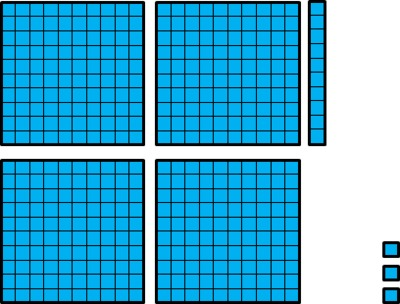Note: pay close attention to the students' illustrations. They may make the 3 in 0.203 one base ten unit less than the 2. In this case, it will appear that 0.203 is greater than 0.21.

HideShow

## Rounding

Lastly, students build on their understanding of rounding, which begins in Grade 3 with rounding whole numbers to the nearest 10 and 100 (3.NBT.A.1) and continues in Grade 4 with rounding multi-digit whole numbers to any place. (4.NBT.A.3) In Grade 5, students are expected to round decimals to any place using place value understanding. (5.NBT.A.4) The inclusion of place value understanding here is important to note; many of us learned to round by memorizing rules or even rhymes (“5 or more, Raise the score, 4 or less, Give it a rest!”). However, memorizing rules without developing understanding is not enduring for most students because these tricks have little substantive meaning, are easy to forget, and are often misapplied. In contrast to memorizing rules, using a number line to teach rounding helps students visualize how numbers are closer to a particular ten or hundred; it is strategy based on students’ existing number sense. While we certainly wouldn’t expect students to draw a number line every time they round for the duration of their mathematical lives, the visual representation helps to form a solid conceptual basis for rounding. The example below asks students to use a number n and determine which number to round it to.

#### Rounding to Tenths and Hundredths

A number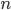is shown on the number line.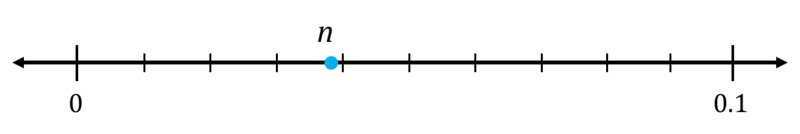1. The tick marks are evenly spaced. Label them.

2. What isrounded to the nearest hundredth?

3. What isrounded to the nearest tenth?

Since students don’t know the exact value of n, they have to use its position on the number line to make a determination. The very fact that the task uses “n” instead of a number serves both to rely on and build conceptual understanding; students can’t apply a simple rule or rhyme to find the answer.

## Powers of 10

A key advancement in Grade 5 is learning the meaning and notation for an exponent as it denotes powers of 10 (e.g., 103 = 10 x 10 x 10). (Work with powers of numbers other than 10 begins in Grade 6. (6.EE.A.1)) Students should understand patterns in the number of 0s in the product when multiplying a whole number by a power of 10, and the patterns in the placement of the decimal point when multiplying or dividing a decimal by a power of 10. Both patterns can be explained using place value understanding. Let’s take a closer look at these patterns; we’ll consider the pattern in the number of 0s first.

### Patterns with 0s

Students know that when multiplying a whole number by 10, each digit in the product is ten times as large and consequently shifts one place to the left (e.g., 71 x 10 = 710). This is because the value of each place in the base-ten system is 10 times as large as the value of the place to its right (e.g., 700 ÷ 70 = 10 and 10 ÷ 1 = 10). In Grade 5, students observe these patterns based on multiplication by powers of 10. For example:

71 x 100 = 71 x 10 x 10 = 71 x 102 = 7100

71 x 1000 = 71 x 10 x 10 x 10 = 71 x 103 = 71,000.

The pattern that emerges with the 0s is that when multiplying a whole number by a power of 10, the product has the same digits as the whole number, which are then followed by the number of 0s denoted by the power of 10. For example, from examples like those above, students will likely predict that, for example, when multiplying 71 x 104 the product will have the digits 7 and 1 followed by four 0s: 710,000. This pattern also accounts for multiplying a multiple of 10 by a power of 10. For example, when multiplying 70 x 104 the product has the digits 7 and 0 followed by four 0s: 700,000.2 Progressions for the Common Core State Standards in Mathematics (draft): Number and Operations in Base Ten, K-5, p. 18. Progressions for the Common Core State Standards in Mathematics (draft): Number and Operations in Base Ten, K-5, p. 18. Progressions for the Common Core State Standards in Mathematics (draft): Number and Operations in Base Ten, K-5, p. 18.

### Placement of the decimal point

With respect to patterns with the placement of the decimal point, students extend the same place value understanding used with whole numbers to decimals: when multiplying a decimal by 10, each digit in the product is 10 times as large and shifts one place to the left, this includes the digits in the places to the right of the decimal point. For example, multiplying 6.421 by 102 is the same as multiplying 6.421 by 10 two times. The digits in the product are 100 times as large and shift two places to the left (e.g., 6.421 x 102 = 6.421 x 10 x 10 = 642.1). The pattern that emerges is that the digits shift to the left by the number of places indicated by the power of 10 (in the case of 102, two places), and the decimal point is positioned that many places to the right (from 6.421 to 642.1). Students should also explore dividing by powers of 10, (i.e., the digits in the quotient are shifted to the right and consequently the decimal point is positioned to the left). Thus making the quotient decreased by the power of 10, so 6.421102 is the same as dividing 6.421 by 10 twice which yields 0.06421. The following task requires students to reason about patterns with 0s and patterns with the decimal point; note again the inclusion of “explain” in this task.

#### Marta’s Multiplication Error

Marta made an error while finding the product 84.15 × 10.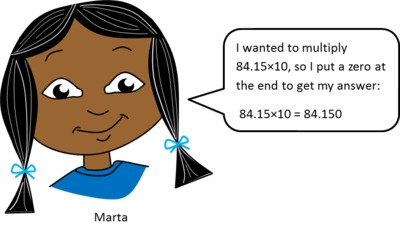This task highlights the common misconception that students have when multiplying a decimal (rather than a whole number) by a power of 10. Students will sometimes append a zero to the right of the decimal point, but not move the decimal point to account for the power of 10, as illustrated by Marta’s response in the task.They think that adding a 0 is sufficient, but forget that the patterns in use are based on place value; appending a zero in this position does not change the value of any of the digits. Students should note that 84.15 x 10 is not 84.150, but actually 841.5, and they should be able to explain why using place value understanding. When appending a 0 to a whole number the value of the number changes because each digit shifts one place to the left. However, when appending a 0 to a decimal the value of the number does not change. As the solution to the task notes, this could also be illustrated using expanded form and the distributive property.

## Operations with whole numbers and decimals

The second cluster in the Number & Operations in Base Ten domain deals with performing operations with whole numbers and decimals. Let’s read the standards associated with this cluster, and then we’ll think through what they mean and how they look in practice.

5.NBT.B | Perform operations with multi-digit whole numbers and with decimals to hundredths.

 5.NBT.B.5 Fluently multiply multi-digit whole numbers using the standard algorithm. 5.NBT.B.6 Find whole-number quotients of whole numbers with up to four-digit dividends and two-digit divisors, using strategies based on place value, the properties of operations, and/or the relationship between multiplication and division. Illustrate and explain the calculation by using equations, rectangular arrays, and/or area models. 5.NBT.B.7 Add, subtract, multiply, and divide decimals to hundredths, using concrete models or drawings and strategies based on place value, properties of operations, and/or the relationship between addition and subtraction; relate the strategy to a written method and explain the reasoning used.

## Multiplication and division with whole numbers

By the end of Grade 5, students are expected to fluently multiply multi-digit whole numbers using the standard algorithm. (5.NBT.B.5) The progression toward this fluency begins in Grade 3 and is rooted in developing meaning for multiplication and division. The example below shows how students might support multi-digit multiplication using an area model and the connection of that area model to the standard algorithm.

#### Grade 5, Module 2, Lesson 6: Concept Development

Problems 2–3

814 × 39

624 × 82

T: (Write 814 × 39 on the board.) Partner A, use the standard algorithm to solve. Partner B, draw an area model to solve.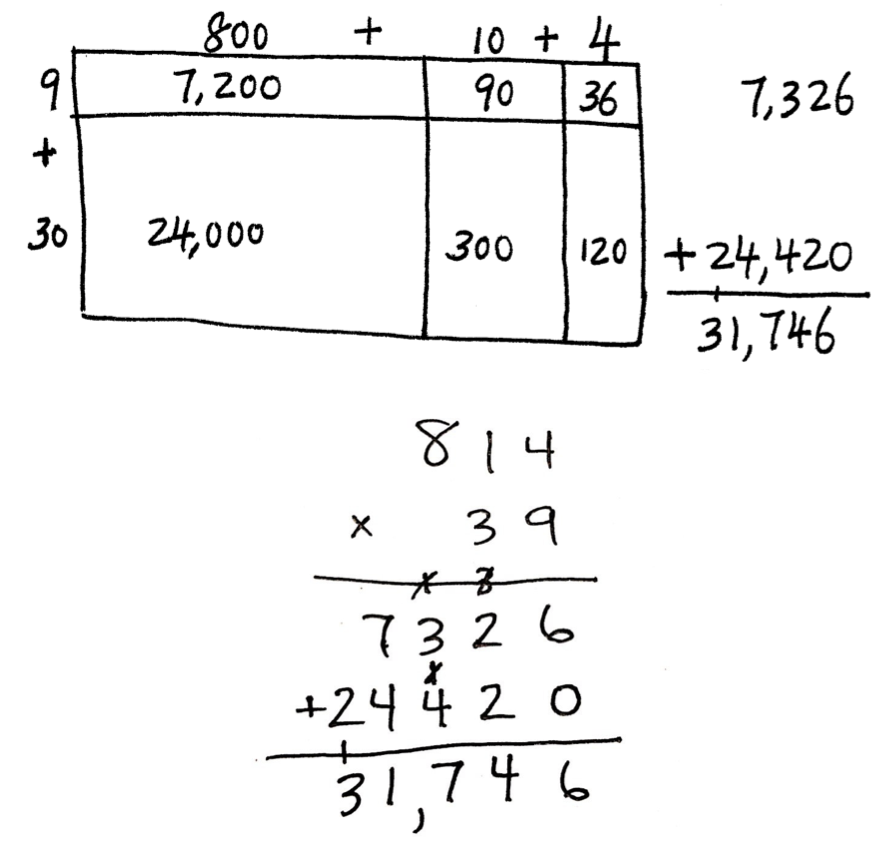T: (Draw and solve.)

Examples like these are an important part of the work in Grade 5; students become proficient with the standard algorithm by connecting it to prior work with visual models and other strategies.

The Standards distinguish strategies from algorithms. Strategies are “chosen for specific problems,” and algorithms are “predefined steps applicable to a class of problems.”3 CCSS Glossary CCSS Glossary CCSS Glossary In Grade 5, students move from work exclusively with strategies (i.e., to multiply 23 by 7, decompose 23 into 20 + 3 and then apply the distributive property to multiply 7 by 20 + 3) that vary based on the problem type to algorithms (i.e., the historically typical “stacking” of numbers) that will work for multiplying any pair of whole numbers.

Also in Grade 5, students extend their work with division of whole numbers to 2-digit divisors (roughly speaking, the number you “divide by”) with up to 4-digit dividends (roughly speaking, the number “being divided”). (5.NBT.B.6) For example, in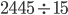, 2445 is the dividend and 15 is the divisor. The expectation is that students divide whole numbers using strategies based on place value, the properties of operations, and the relationship between multiplication and division. They illustrate and explain their work using equations, rectangular arrays, and/or area models. This builds from Grade 4, where students divide with 1-digit divisors and up to four-digit dividends. (4.NBT.B.6) Like Grade 4, students in Grade 5 decompose the number being divided into base-ten units and find the quotient for each unit, starting from the largest unit (e.g., 31010 = 3 hundreds and 1 ten divided by 10, leading to 3 tens and 1 one (31) as the quotient).4 Progressions for the Common Core State Standards in Mathematics (draft): Number and Operations in Base Ten, K-5, p. 18. Progressions for the Common Core State Standards in Mathematics (draft): Number and Operations in Base Ten, K-5, p. 18. Progressions for the Common Core State Standards in Mathematics (draft): Number and Operations in Base Ten, K-5, p. 18. Eventually, students relate this work to the standard algorithm. The lesson below introduces students to division with two-digit divisors by using a dividend and divisor that are multiples of ten. Since students are familiar with multiplying and dividing by 10, this is a comfortable place to begin thinking about division by two-digit numbers.

#### Grade 5, Module 2, Lesson 16: Concept Development

Problem 1: 420 ÷ 10

T: (Write 420 ÷ 10 horizontally on board.) Let’s use place value disks to solve this problem. Work with a partner to show 420 using the disks.

T/S: (Draw 4 hundred disks and 2 ten disks, as shown to the lower right.)

T: Say 420 in unit form.

S: 4 hundreds 2 tens.

T: Let’s divide. What is 1 hundred divided by 10?

S: 10.

T: If 1 hundred divided by 10 is 1 ten, what is 4 hundreds divided by 10?

S: 4 tens.

T: I’ll show that division with my place value disks. You do the same. (Draw an arrow showing ÷ 10 and 4 tens disks.)

S: (Draw.)

T: What is 1 ten divided by 10?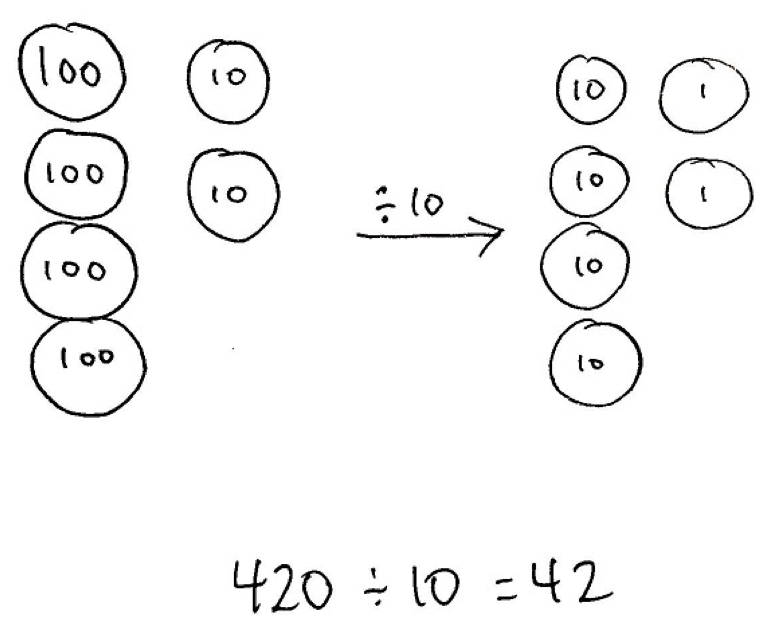S: 1.

T: If 1 ten divided by 10 is 1 one, what is 2 tens divided by 10?

S: 2 ones.

T: Show that division with place value disks.

T: (Point to the original problem.) Read the division sentence with the solution.

S: 420 ÷ 10 = 42.

T: Let’s solve this problem again using our place value charts. Show 420 in numerical form on your chart.

S: (Write 420 on the place value chart.)

T: When we divide this whole number by 10, will the quotient be greater than or less than 420?

S: Less than 420.

T: Therefore, in which direction will the digits shift when we divide by 10?

S: To the right.

T: How many places to the right?

S: One place to the right.

T: Use arrows to show the shifting of digits. Show your neighbor when you’re finished, and then discuss whether this happens every time we divide a number by 10.

S: (Work and share.)

T: Say the division sentence, or the division equation, you just completed on your place value chart.

S: 420 ÷ 10 = 42.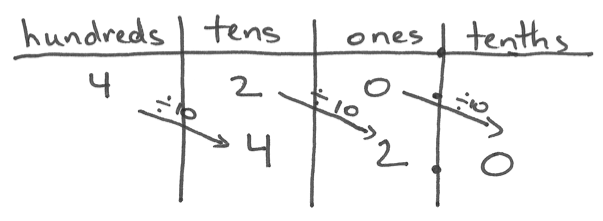HideShow

In this example, students decompose 420 into base-ten units (4 hundreds and 2 tens). They use place value disks (and the place value chart) to show the division of each base-ten unit by the divisor (10). In subsequent lessons, students use rounding (of both the dividend and the divisor) to estimate the partial quotients, relate their estimates to the standard algorithm, and then check their work with multiplication (and addition with respect to remainders). Note that while students might begin to relate multi-digit division to the standard algorithm, fluency with the standard algorithm for division is not expected until Grade 6.6.NS.B.6 Rushing to teach the algorithm, absent connection to conceptual understanding, may cause confusion for students because memorizing a procedure without understanding why it works does not support long term retention of the mathematics.So as much as you want to jump to significant practice with the standard algorithm, don’t! Spend time practicing solving division problems using place value strategies, the properties of operations, and the relationship between multiplication and division demonstrating how they connect to the standard algorithm adequately in Grade 5, so students can then apply it fluently in Grade 6. The following example shows use of the standard algorithm with connection to place-value understanding:

#### Grade 5, Module 2, Lesson 22: Concept Development

Problem 1: 590 ÷ 17

T: (Write 590 ÷ 17 in the algorithm on the board.) Can we divide 5 hundreds by 17?

S: Not without regrouping.

T: Let's work with 59 tens, then. We can divide 59 tens into 17 groups or groups of 17. Tell me how to estimate to divide 59 tens by 17.

S: 60 tens ÷ 20 = 3 tens

T: Record 3 tens, and find the remainder in the tens place. 3 tens times 17 is?

S: 51 tens.

T: (Record 51 tens below 59 tens.) Remind me why we record here. (Point to the algorithm.)

S: We record the 5 in the hundreds place and the 1 is in the tens place because we know 51 tens is the same as 510.

T: How many tens are remaining?

S: 8 tens.

T: Can we divide 8 tens by 17?

S: Not without regrouping.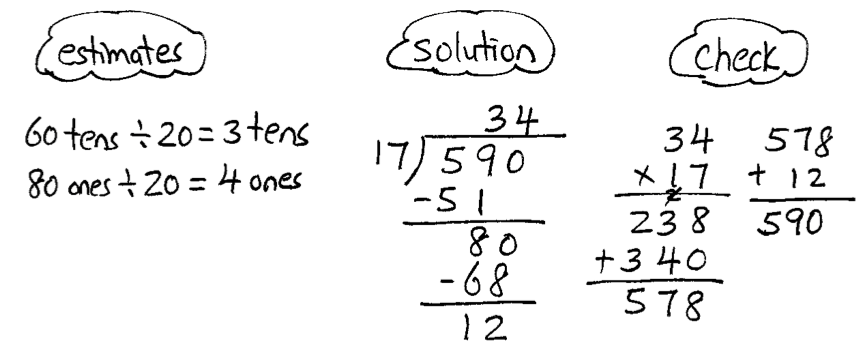T: We need to decompose these 8 tens into 80 ones. There are no ones in the whole to add in.

(Point to the zero in the ones place of the whole.)

T: Now, we have 80 ones divided by 17. Tell me how to divide 80 by 17?

S: 80 ones ÷ 20 = 4 ones.

T: Record 4 ones in the quotient. What is 17 × 4 ones?

S: 68 ones.

T: What is 80 – 68? How many ones remain?

S: 12.

T: Could we make another group of 17?

S: No.

T: What is our quotient?

S: 34, remainder 12.

T: What is 34 units of 17 plus 12 ones?

S: 590.

HideShow

In the lesson above, multiplying two-digit numbers by one-digit numbers (e.g., 3 x 17 and 4 x 17) should be quick mental computations for students in Grade 5, because in Grades 3 and 4, students work extensively with the distributive property as a strategy to multiply. In this case, 3 x 17 = 3 x (10 + 7) = (3 x 10) + (3 x 7) = 30 + 21 = 51.

## Operations with decimals

Students use place value understanding to perform all four operations with decimals to hundredths. (5.NBT.B.7) Students perform these operations by applying the same conceptual strategies they use with whole numbers and by using concrete models and drawings. Similarly, they compose and decompose decimals in the same way they do with whole numbers. Fluency with standard algorithms for operations with decimals is not expected until Grade 6. (6.NS.B.3)

### Addition and subtraction with decimals

Many of us grew up learning that we must “line up” the decimal points when adding and subtracting decimals. In Grade 5, the Standards require us to make sure students understand why; students must be able to connect strategies to written methods and explain the reasoning used. (5.NBT.B.7) The following example shows how students use a place value chart to decompose and compose decimals in order to subtract. The chart strategy is connected to a written method that looks similar to the standard algorithm for subtraction.

#### Grade 5, Module 1, Lesson 10: Concept Development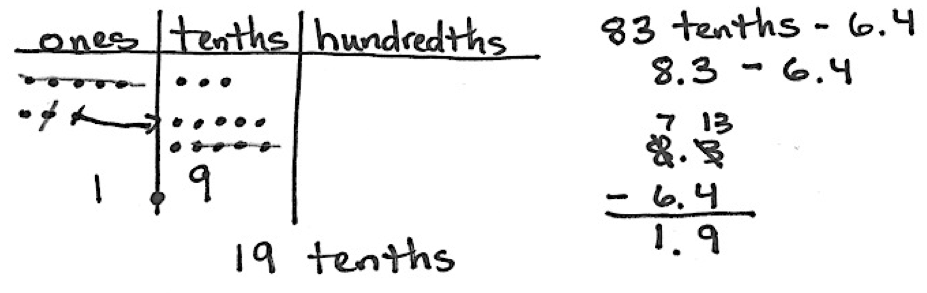The place value chart shows regrouping of a one into 10 tenths and then students subtract like units from like units, which aligns the decimal point. To further emphasize this point we might ask students to explain why the decimal points “line up” when subtracting in this example. Students should be able to explain that the decimal points line up because we need to subtract like units (i.e., tenths from tenths, and ones from ones), which is the same approach taken when we add and subtract whole numbers.

### Multiplication and division with decimals

Methods for multiplying and dividing whole numbers can be applied to multiplying and dividing decimals. In the following example, students multiply a decimal by a whole number using a place value chart and then relate the place value strategy to an area model and written method.

#### Grade 5, Module 1, Lesson 11: Concept Development

T: (Write 4 0.423 = _______ on the board.) Solve by drawing on your place value chart.

S: (Solve.)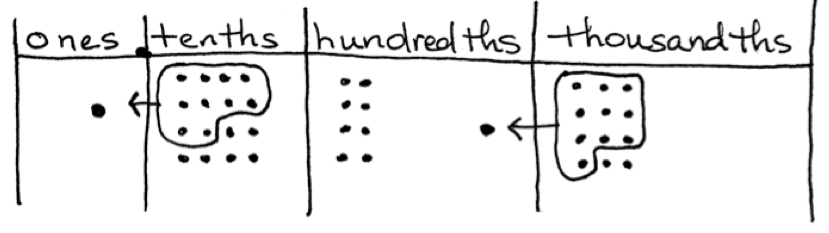S: 1 and 692 thousandths.

S: 4 times 3 thousandths is 12 thousandths, so we had to bundle 10 thousandths to make 1 hundredth.

T: Did any other units have to be regrouped?

S: The units in the tenths place. Four times 4 tenths is 16 tenths, so we had to regroup 10 tenths to make 1 whole.

T: Let’s record what happened using an area model and an equation showing the partial products.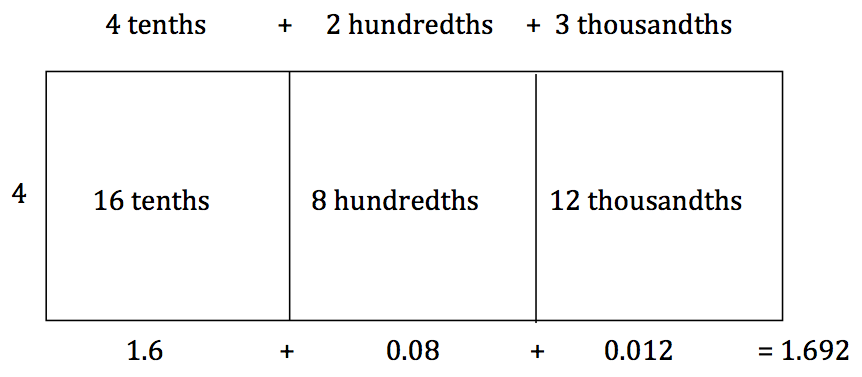In Grade 5, students should also multiply decimals by decimals. They can multiply decimals to the hundredths, however, since there is focus on decimals to the thousandths, (5.NBT.A.3) the products should not extend beyond the thousandths. Similarly with division, students should divide decimals to the hundredths, and the quotients should not extend beyond the thousandths place; students should divide decimals by whole numbers as well as by decimals. In the example below, students are asked to divide a decimal by a decimal.

#### Grade 5, Module 4, Lesson 30: Problem Set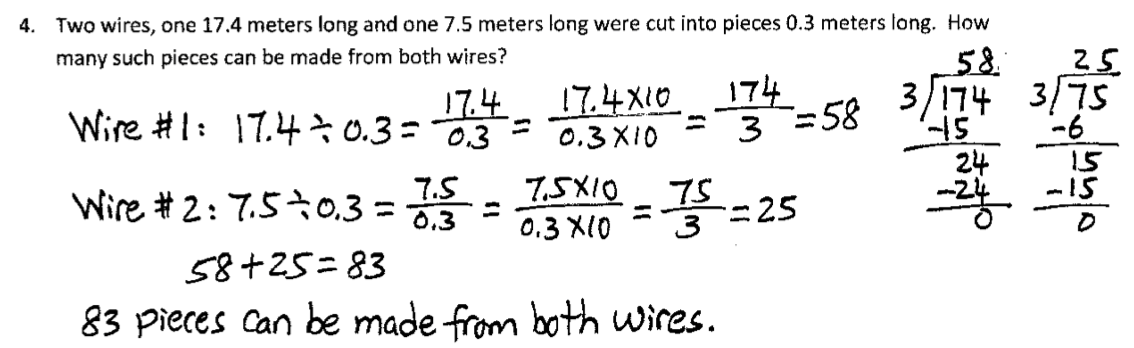In this example, students divide a decimal by a decimal divisor by writing the division problem as a fraction and then multiplying the fraction by 10/10 in order to yield an equivalent fraction with a whole number in the denominator. Students then relate the resulting fraction to the division algorithm. Note this kind of reasoning relies on important prerequisite understandings of fractions: understanding fractions as division, (5.NF.B.3) multiplication as scaling, (5.NF.B.5.B) and fraction equivalence. (4.NF.A.)1

## Using the four operations in problem-solving

In Grade 5, students are expected to solve problems using the four operations. There are various problem types that students should engage with while using grade-appropriate numbers. These problem types have the unknown in various positions and can range from one-step to multi-step. Table 2 below comes from the Standards, and describes the common multiplication and division situations students should master in Grades 3-5. In Grade 5, students should have experience with these varying situations using multi-digit whole numbers as well as decimals and fractions. It will be helpful to spend some time digging into all of the different problem situations shown in the table as we will refer to them later.

Many of us have learned that solving word problems involves finding “key words,”—words like “more” and “total” tell us to add, while words like “fewer” and “less” tell us to subtract. But what about a problem like this:

Lucy has six fewer apples than Julie. Lucy has eight apples. How many apples does Julie have?5 Adapted from CCSSM Table 2. Adapted from CCSSM Table 2. Adapted from CCSSM Table 2.

The “key word” in this problem, “fewer,” actually hints at the wrong operation; subtracting will not result in the correct answer.

A better way to help students with problem-solving is to help them think situationally about the varied contexts. Using word problems to create meaning for operations helps students to better understand how to apply operations.

Common multiplication and division situations (1)

 UNKNOWN PRODUCT GROUP SIZE UNKNOWN (“HOW MANY IN EACH GROUP?” DIVISION) NUMBER OF GROUPS UNKNOWN (“HOW MANY GROUPS?” DIVISION) 3 x 6 = ? 3 x ? = 18, and 18 ÷ 3 = ? ? x 6 = 18, and 18 ÷ 6 = ? EQUAL GROUPS There are 3 bags with 6 plums in each bag. How many plums are there in all? Measurement example. You need 3 lengths of string, each 6 inches long. How much string will you need altogether? If 18 plums are shared equally into 3 bags, then how many plums will be in each bag? Measurement example. You have 18 inches of string, which you will cut into 3 equal pieces. How long will each piece of string be? If 18 plums are to be packed 6 to a bag, then how many bags are needed? Measurement example. You have 18 inches of string, which you will cut into pieces that are 6 inches long. How many pieces of string will you have? ARRAYS (2), AREA (3) There are 3 rows of apples with 6 apples in each row. How many apples are there? Area example. What is the area of a 3 cm by 6 cm rectangle? If 18 apples are arranged into 3 equal rows, how many apples will be in each row? Area example.A rectangle has area 18 square centimeters. If one side is 3 cm long, how long is a side next to it? If 18 apples are arranged into equal rows of 6 apples, how many rows will there be? Area example. A rectangle has area 18 square centimeters. If one side is 6 cm long, how long is a side next to it? COMPARE A blue hat costs \$6. A red hat costs 3 times as much as the blue hat. How much does the red hat cost? Measurement example. A rubber band is 6 cm long. How long will the rubber band be when it is stretched to be 3 times as long? A red hat costs \$18 and that is 3 times as much as a blue hat costs. How much does a blue hat cost? Measurement example. A rubber band is stretched to be 18 cm long and that is 3 times as long as it was at first. How long was the rubber band at first? A red hat costs \$18 and a blue hat costs \$6. How many times as much does the red hat cost as the blue hat? Measurement example. A rubber band was 6 cm long at first. Now it is stretched to be 18 cm long. How many times as long is the rubber band now as it was at first? GENERAL a x b = ? a x ? = p and p ÷ a = ? ? x b = p, and p ÷ b = ?

(1) The language in the array examples shows the easiest form of array problems. A harder form is to use the terms rows and columns: The apples in the grocery window are in 3 rows and 6 columns. How many apples are in there? Both forms are valuable.

(2) Area involves arrays of squares that have been pushed together so that there are no gaps or overlaps, so array problems include these especially important measurement situations.

(3) The first examples in each cell are examples of discrete things. These are easier for students and should be given before the measurement examples.

CCSSM Table 2.

Table 1 in the Standards describes the various addition and subtraction problem types appropriate for elementary school.6 CCSSM Table 1. CCSSM Table 1. CCSSM Table 1. It is important that students engage with the different problem situations in Table 1 using multi-digit whole numbers, decimals, and fractions. Though these tables describe one-step problems, students should have lots of opportunity to engage with two-step and multistep problems using any combination of the four operations. In Grade 5, students should continue practice with representing problems with equations and using a letter to represent the unknown. The example below shows a two-step division problem leading to a decimal as the quotient.

#### Grade 5, Module 2, Lesson 28: Concept Development

Ava is saving for a new computer that costs \$1,218. She has already saved half of the money. Ava earns \$14.00 per hour. How many hours must Ava work to save the rest of the money?

This two-step equal groups with number of groups unknown problem is a step forward for students as they divide the total in half and use their decimal division skills to divide 609 by 14 to find the number of hours Ava needs to work. In this case, the divisor represents the size of the unit. While circulating, use professional judgment to look for other alternate modeling strategies that can be quickly mentioned or explored more deeply.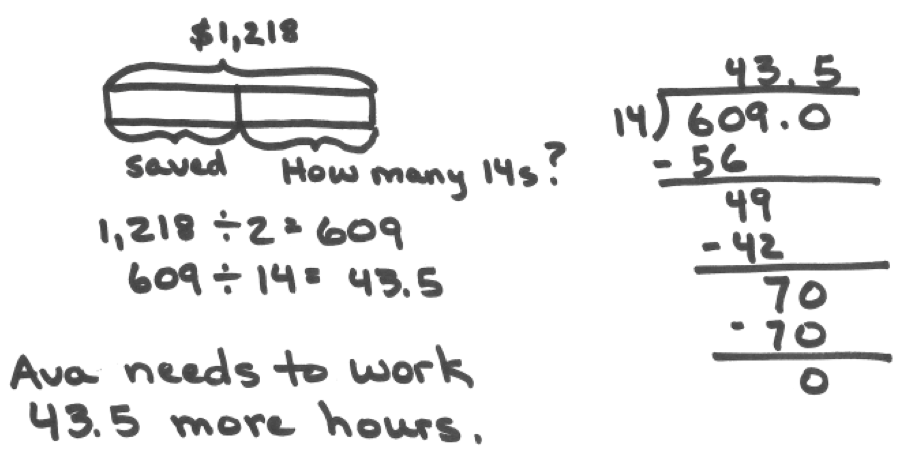This is an example of a two-step equal groups problem in which the number of groups (i.e., the number of hours) is unknown. First, the students have to determine how much money Ava has yet to earn (\$609). Then students divide 609 by 14 to find the number of hours. Note, dividing 609 by 14 requires them to move beyond finding a remainder and to reason about the placement of the decimal point in order to divide 70 tenths by 14 to get 5 tenths.

# Part 2: How do Number & Operations in Base Ten relate to other parts of Grade 5?

There are lots of connections among standards in Grade 5; if you think about the standards long enough, you’ll probably start to see these relationships everywhere.7 The idea that standards relate strongly to one another is known as coherence, and is a distinctive feature of the Common Core State Standards for Math. If you’re interested in exploring more of the connections between standards, you might want to check out the Student Achievement Partners Coherence Map, which illustrates them visually. The idea that standards relate strongly to one another is known as coherence, and is a distinctive feature of the Common Core State Standards for Math. If you’re interested in exploring more of the connections between standards, you might want to check out the Student Achievement Partners Coherence Map, which illustrates them visually. The idea that standards relate strongly to one another is known as coherence, and is a distinctive feature of the Common Core State Standards for Math. If you’re interested in exploring more of the connections between standards, you might want to check out the Student Achievement Partners Coherence Map, which illustrates them visually. In this section, we’ll talk about the connection between the standards in the Number & Operations in Base Ten (NBT) domain and the standard in the first cluster of the Measurement & Data (MD) domain. The standard in this MD cluster is a supporting standard and can be used to support work with the NBT standards.

5.MD.A | Convert like measurement units within a given measurement system.

 5.MD.A.1 Convert among different-sized standard measurement units within a given measurement system (e.g., convert 5 cm to 0.05 m), and use these conversions in solving multistep, real world problems.

This standard builds on work with conversions in Grade 4, where students convert from larger to smaller units. (4.MD.A.1) In Grade 5, students build on this understanding to convert measurements from a smaller unit to a larger unit. Students relate the idea that a digit in one place is 1/10 the size of the digit in the place to its left (5.NBT.A.1 )to the idea that smaller metric units are 1/10, 1/100, or 1/1000 the size of larger units. The following lesson gives an example of this relationship.

#### Grade 5, Module 1, Lesson 4: Application

T: Here is a place value chart. (Show the place value chart from thousands to thousandths without other headings.)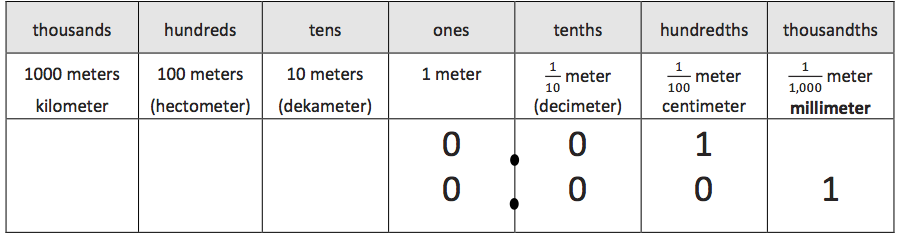T: Here is a set of column headings based on metric length related to our place value chart, designating one meter as the base unit, or the ones place.

T: Use your meter strip to show and explain to your partner the lengths that relate to the tenths, hundredths, and thousandths places. (Move through the tenths, hundredths, and thousandths until identifying and naming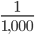meter as 1 millimeter.)

Understanding the relationship between the metric units and the place value chart helps students know whether they are moving from a larger unit to a smaller unit or the reverse. It also illustrates the multiplicative relationship between units.

Since this is a supporting cluster, students use conversions to solve multistep, real-world measurement problems in order to support addition, subtraction, multiplication, and division with whole numbers, decimals, and fractions. This builds from work in Grade 4 where students engage with measurement contexts involving whole numbers and simple fractions and decimals. (4.MD.A.2) The contexts for the multistep problems should involve measurement systems the students are familiar with: distance, intervals of time, liquid volumes, masses of objects, and money. The example below involves converting from a smaller unit to a larger unit.

#### Minutes and Days

What time was it 2011 minutes after the beginning of January 1, 2011?

Solution

January 1, 2011 begins at 12:00 AM. To find the time 2011 minutes later will require changing units since time is told in hours and minutes. There are 60 minutes in an hour so to see how many hours there are in 2011 minutes we can perform the division problem 2011 ÷ 60. Since 30 × 60 = 1800, we can write

2011 = 30 × 60 + 211.

Next, there are three groups of 60 in 211, with a remainder of 31 so we get

2011 = 30 × 60 + 3 × 60 + 31.

Using the distributive property this last expression is equivalent to

2011 = (30 + 3) × 60 + 31.

So 2011 minutes is the same as 33 hours and 31 minutes. Now 33 hours is one day and an additional 9 hours so this means that 2011 minutes is one day, nine hours, and thirty-one minutes. So 2011 minutes after the beginning of 2011 it is January 2 and it is 9:31 AM.

In this task, students divide a 4-digit number by a two-digit number to convert hours to minutes (2011 ÷ 60). Alternatively, as shown in the solution, students can use multiplication to solve the problem.

# Part 3: Where do the Number & Operations in Base Ten Standards come from, and where are they going?

Podcast clip: Importance of Coherence with Andrew Chen and Peter Coe (start 9:34, end 26:19)

## Where does work with place value and operations in Grade 5 come from?

### Grades 3-4: Multiplication and division with whole numbers

• Developing meaning for these operations with work on equal groups, arrays, and area, along with representing problems with models, drawings, and equations. (3.OA.A.3, 3.MD.C.7)
• Using strategies for multiplication and division based on place value, properties of operations (commutative, associative, and distributive), and the relationship between multiplication and division (e.g., understanding that a division problem can be thought of as an unknown factor problem). (3.OA.B.5, 3.OA.B.6)

The example below illustrates two different solution methods for an equal groups problem.

#### Grade 3, Module 1, Lesson 12: Application Problem

A chef arranges 4 rows of 3 red peppers on a tray. He adds 2 more rows of 3 yellow peppers. How many peppers are there altogether?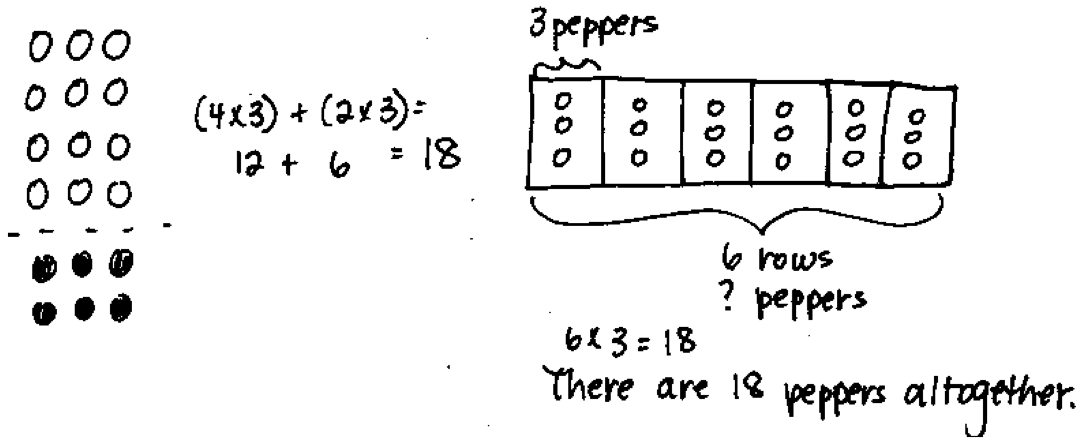Note: Students might solve using an array to model the distributive property (Lesson 10) or a tape diagram (Lesson 11). If they use the latter strategy, it is likely their first use of a tape diagram to solve multiplication. The problem is a review that provides an exploratory opportunity for students to select and use appropriate tools.

As noted, students might use the distributive property or a tape diagram to represent the problem. The distributive property is introduced to develop fluency with single-digit facts and students continue to apply it with multi-digit numbers.

In Grade 4, students develop a new understanding of multiplication as a comparison. Multiplicative comparison involves comparing quantities multiplicatively instead of additively, which students have been working on since Grade 1. (1.OA.A.1)

In additive comparisons, the underlying question is: What amount would be added to one quantity in order to result in another? By contrast, in multiplicative comparisons, the underlying question is: What factor would be multiplied by one quantity in order to result in the other? The example below illustrates this distinction.

 Multiplicative comparison “My friend has 2 apples and I have 10 apples, so I have 5 times as many apples as she does.” 2 × ___ = 10 Additive comparison “My friend has 2 apples and I have 10 apples, so I have 8 more apples than she does.” 2 + ___ = 10

Students learn to make the distinction between multiplicative and additive comparisons by engaging with various types of multiplicative comparison word problems. (4.OA.A.2) The task below shows some problems involving multiplicative comparison.

#### Comparing Money Raised

1. Helen raised \$12 for the food bank last year and she raised 6 times as much money this year. How much money did she raise this year?

1. Sandra raised \$15 for the PTA and Nita raised \$45. How many times as much money did Nita raise as compared to Sandra?

1. Luis raised \$45 for the animal shelter, which was 3 times as much money as Anthony raised. How much money did Anthony raise?

Similarly, more experience with additive comparison problems (e.g., with multi-digit whole numbers (4.NBT.B.4)) is necessary to clarify the distinction between multiplicative and additive comparison. In Table 2 from the Standards (see Part 1), the compare problems highlight the different multiplicative comparison situations students should encounter.9 CCSSM Table 2. CCSSM Table 2. CCSSM Table 2.

Also in Grade 4, students multiply and divide with larger numbers. They continue to multiply and divide using strategies based on place value, the properties of operations, and the relationship between multiplication and division. (4.OA.A.2,4.NBT.B.5, 4.NBT.B.6) And they continue to use visuals like area models, connecting them to equations and written computation methods, which provides meaning for algorithms. The example below shows how a student might represent multi-digit multiplication using a model that supports a written method typical in Grade 4.

#### Grade 4, Module 3, Lesson 8: Application Problem

Andre buys a stamp to mail a letter. The stamp costs 46 cents. Andre also mails a package. The postage to mail the package costs 5 times as much as the cost of the stamp. How much does it cost to mail the package and letter?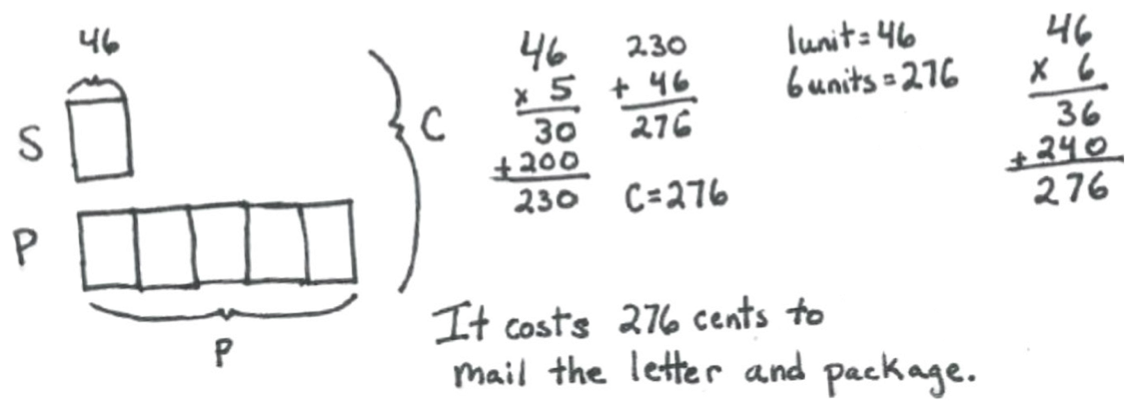Note: This problem is a review of Lesson 7 and incorporates multiplicative comparison. Students who examine the tape diagram find a more rapid solution is to multiply to find 6 units of 46 cents.

The example shows a two-step problem involving multiplicative comparison and addition. It shows the use of place value strategies to compute partial products when multiplying a two-digit number by a one-digit number.

Some students in Grade 4 may progress from written methods that display all calculations for multiplication and division (e.g., partial products) to more compact written methods. The example below illustrates how different strategies relate to the standard algorithm.

#### Grade 4, Topic C Overview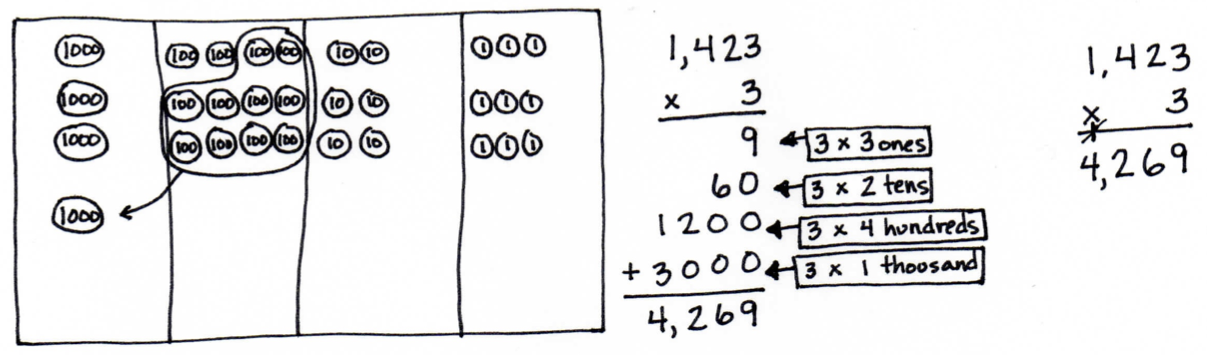Grade 4, Module 3, Topic C Overview Available from engageny.org/resource/grade-4-mathematics-module-3-topic-c-overview; accessed 2015-05-29. Copyright © 2015 Great Minds. UnboundEd is not affiliated with the copyright holder of this work.

#### Grade 3, Module 2, Lesson 8: Problem Set

1. Use tape diagrams to model the following problems. Keiko and her brother Jiro get weighed at the doctor’s office. Keiko weighs 35 kilograms, and Jiro weighs 43 kilograms.

1. What is Keiko and Jiro’s total weight?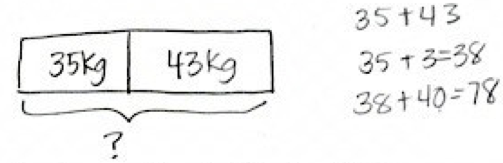Keiko and Jiro weigh ____78____ kilograms.

1. How much heavier is Jiro than Keiko?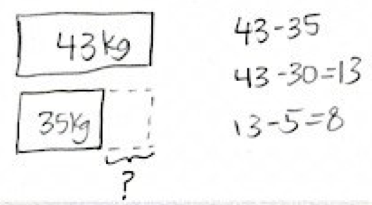Jiro is ___8___ kilograms heavier than Keiko.

With larger numbers, it becomes more efficient to use more compact written methods. The following example from Grade 4 illustrates how students might relate place value models to the standard algorithm for addition for multi-digit numbers. Notice the composing of a hundred from 10 tens and that it is recorded on the addition line.

#### Grade 4, Module 1, Lesson 11: Concept Development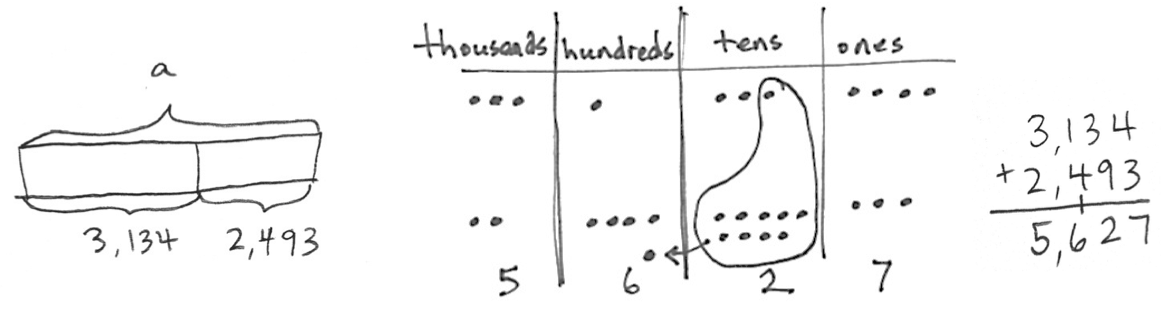### Grades K-4: Application of the four operations

In Grade 4, students engage in solving multistep word problems using the four operations. (4.OA.A.3) This is a culminating standard for a sequence beginning in Kindergarten. (K.OA.A.2, 1.OA.A.1, 2.OA.A.1, 3.OA.D.8) More specifically, students in Grade 2 began working with two-step problems that were limited to addition and subtraction, and expanded to include multiplication and division in Grade 3. In Grade 4, the problems are multistep, generally with no more than three steps, and can include any combination of the four operations with whole numbers and integrate concepts from MD standards.

The following examples illustrate different kinds of multistep word problems in Grade 4. In the first example, students are expected to solve one problem that involves several steps in order to determine the total amount of money earned for both days.

#### EngageNY Grade 4, Module 3, Lesson 7: Application Problem

The basketball team is selling T-shirts for \$9 each. On Monday, they sold 4 T-shirts. On Tuesday, they sold 5 times as many T-shirts as on Monday. How much money did the team earn altogether on Monday and Tuesday?This solution is modeled using different kinds of representations. The step involving multiplicative comparison is modeled with a tape diagram, and labeled equations are used to show the multiplication.

Though the prior example was a single, extended task, multistep problems can also comprise a series of questions developing from a particular context, as in the next example. Students have to pay close attention to the language to determine whether the relationship being asked about is additive or multiplicative. Given that there are so many questions, the size of the numbers is restricted.

#### Carnival Tickets

Every year a carnival comes to Hallie's town. The price of tickets to ride the rides has gone up every year.1. In 2008, Hallie’s allowance was \$9.00 a month. How many carnival tickets could she buy with one month's allowance?

1. If her allowance had stayed the same, \$9.00 a month, how many carnival tickets could she buy in 2012?

1. In 2012, Hallie’s allowance was \$14.00 per month. How much did her monthly allowance increase between 2008 and 2012?

1. How much more did a carnival ticket cost in 2012 than it did in 2008?

1. Was Hallie able to buy more carnival tickets in 2008 or in 2012 with one month's allowance?

1. What would Hallie's allowance need to be in 2012 in order for her to be able to buy as many carnival tickets as she could in 2008?

1. What happens to your ability to buy things if prices increase and your allowance doesn’t increase?

HideShow

Notice that in part a, students have to interpret the meaning of a remainder, which is a new understanding in Grade 4. (4.OA.A.3) It’s important to emphasize that we want students to be able to interpret the remainder, rather than simply compute quotients that happen to have remainders.

### Grade 4: Generalizing place value

Students begin thinking about place value in Kindergarten when they observe that the teen numbers are composed of 10 ones and some more ones. (K.NBT.A.1) Students formalize their understanding of the tens place in first grade when they bundle 10 ones to make a ten and that 20 is 2 bundles of ten, or 2 tens and so on. (1.NBT.B.2) In Grade 2, they bundle 10 tens to make a hundred and that 200 is 2 bundles of a hundred or 2 hundreds and so on. (2.NBT.A.1) Understanding place value in K-2 always begins with concrete representation so students can conceptualize the relationship between ones, tens, and hundreds. Students continue to work with numbers within 1000 in Grade 3. (3.NBT.A.2) Students expand their understanding of whole numbers in Grade 4 to numbers greater than 1000. As in in K-2, we want students to understand the relationship between each place value. Specifically, we want them to understand the multiplicative relationship. (4.NBT.A.1) As in previous grades this understanding should be explored concretely and pictorially (i.e., with drawings and manipulatives) before moving on to abstract representations.

In the example below from Grade 4, students show their understanding of place value concepts through use of a place value chart to multiply numbers by 10. This work lays the foundation for further work with place value in Grade 5. (5.NBT.A.1)

#### Grade 4, Module 1, Lesson 1: Problem Set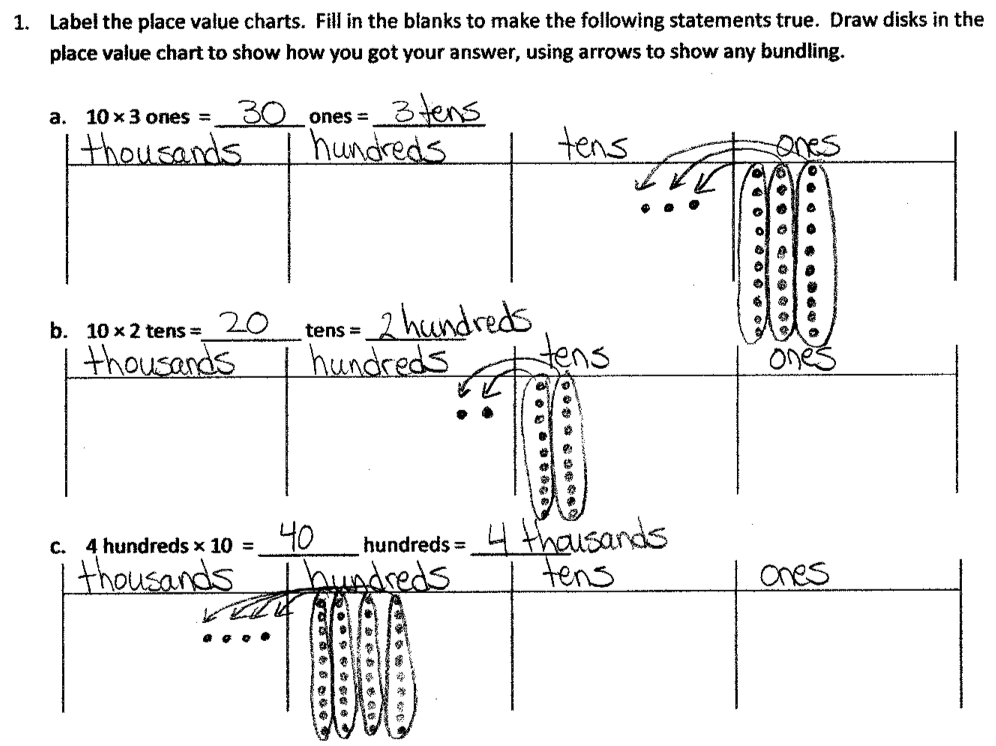Grade 4: Understanding decimal notation for fractions

Students begin their work with decimals in Grade 4 through their work with fractions. (4.NF.C.5, 4.NF.C.6, 4.NF.C.7) Number lines and tape diagrams can be useful tools for helping students relate decimal notation to fractions.

#### Grade 4, Module 6, Topic A: Overview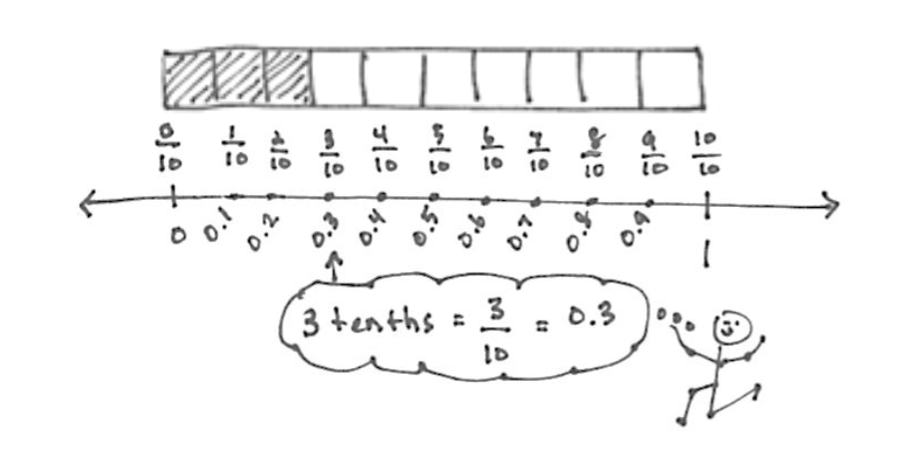As students gain traction with understanding decimal notation for simple fractions, they begin to synthesize what they know about fractions and decimals. Students are then able to more fluently express decimal notation for fractions. The following example shows how students compare fractions to decimals after spending considerable time working on the skill in Grade 4.

#### Grade 4, Module 6, Lesson 11: Problem Set

3. In the long jump event, Rhonda jumped 1.64 meters. Mary jumped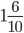meters. Kerri jumped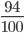meter. Michelle jumped 1.06 meters. Who jumped the farthest?This work, plus what students already know about place value concepts, are the foundation for them to perform operations with multi-digit whole numbers and with decimals to hundredths in Grade 5. (5.NBT.B.7)

### Suggestions for students who are below grade level

If students come to Grade 5 without a solid grasp of the ideas named above (or haven’t encountered them at all), what can you do? It’s not practical (or even desirable) to reteach everything students should have learned in Grades 3-4; there’s plenty of new material in Grade 5, so the focus needs to be on grade-level standards. At the same time, there are strategic ways of wrapping up “unfinished learning” from prior grades. Here are a few suggestions for adapting your instruction to bridge the gaps with respect to preparedness for multiplication and division with whole numbers.

• If a significant number of students struggle to grasp whole number or decimal operations, consider a few days of instruction that start with simpler addition, subtraction, multiplication and division examples (4.NBT.B). In this lesson students can practice adding multi-digit whole numbers. If subtraction is the issue, this topic has lessons that will help students practice subtracting multi-digit whole numbers. This topic has a series of lessons that will help students who require additional practice using place value strategies to multiply multi-digit numbers. You can also check here for lessons that focus on division of multi-digit whole numbers. Consider explicitly relating whole number operations (via a warm-up activity or other example) to decimal operations.
• If a significant number of students don’t have an understanding of place value, you could plan a few lessons that address the foundations of the meaning of each place and how they are related by multiplication (4.NBT.A.1) prior to fifth grade level instruction. Topic A of this Module provides an opportunity for students to practice manipulating and representing the place value of whole numbers. If lessons seem too much, consider a warm-up activity each day that requires students to identify place value in numerals and explain the relationships between places.
• If a significant number of students don’t have conceptual understanding of decimals, consider a few days of instruction that relate decimals to fractions and visual models (4.NF.C). This module will help students make the connection between what they know about fractions and relate that to decimals.

## Beyond Grade 5: What’s next with the four operations?

### Grades 6-7: The number system

It’s helpful to understand how students will build on their understanding of the four operations in later grades. Understanding the progression of this content can help to solidify the focus in Grade 5. It helps us to define the limits of our instruction and to see why we focus so intently on a small number of concepts in Grade 5. At the same time, we realize that we want to set our students up for future success, and knowing the next steps in their journey can help us focus our lessons on the knowledge and skills that matter most.

Having strong conceptual understanding of the four operations is really important to success with middle school mathematics. In Grade 6, students are expected to fluently divide multi-digit whole numbers using the standard algorithm. (6.NS.B.2) And fluency is expected with all four operations with decimals using standard algorithm. (6.NS.B.3) In Grade 7, students are expected to solve real-world and mathematical problems using the four operations with rational numbers. (7.NS.A.3) The examples below illustrate work with decimals in Grade 6 and rational numbers in Grade 7.

 Grade 7 Task A water well drilling rig has dug to a height of –60 feet after one full day of continuous use. a. Assuming the rig drilled at a constant rate, what was the height of the drill after 15 hours? b. If the rig has been running constantly and is currently at a height of –143.6 feet, for how long has the rig been running? (Source: “Drill Rig” by Illustrative Mathematics is licensed under CC BY 4.0.) ➔ This task involves multiplication and division with rational numbers. It also incorporates proportional reasoning, which is an important part of middle school mathematics.

Student Achievement Partners: Focus Grade 5

Draft K-5 Progression on Number and Operations in Base Ten

EngageNY: Grade 5 Module 1 Materials

# Endnotes

 The Common Core State Standards for Mathematics (CCSSM) are organized into major, additional, and supporting clusters in the Focus by Grade Level documents from Student Achievement Partners.

 Progressions for the Common Core State Standards in Mathematics (draft): Number and Operations in Base Ten, K-5, p. 18.

 Progressions for the Common Core State Standards in Mathematics (draft): Number and Operations in Base Ten, K-5, p. 18.

 Adapted from CCSSM Table 2.

 CCSSM Table 1.

 The idea that standards relate strongly to one another is known as coherence, and is a distinctive feature of the Common Core State Standards for Math. If you’re interested in exploring more of the connections between standards, you might want to check out the Student Achievement Partners Coherence Map, which illustrates them visually.

 There are foundational skills and experiences presented in Grade 2. For example, students begin to work with equal groups and arrays and use repeated addition to find the total. (2.OA.C.4 )You can read more about foundations with multiplication and division in Part 3 of the Grade 3 Operations & Algebraic Thinking Content Guide.

 CCSSM Table 2.

# FAQs

## 1. What is a Content Guide?

Our goal in creating the Content Guides has been to provide busy teachers with a practical and easy-to-read resource on what the grade-level math standards are saying, along with examples of instructional materials that support conceptual understanding, problem-solving, and procedural skill and fluency for students.

It’s important to note that content guides are not meant to serve as a curriculum (or any kind of student-facing document), a guide or source material for test-preparation activities, or any kind of teacher evaluation tool.

## 2. What’s in a Content Guide?

Each Content Guide is focused on a specific group of standards. Most Content Guides follow the same three-part structure:

• Part 1 makes clear the student skills and understandings described by this group of standards. This section illustrates the standards using multiple student tasks from freely available online sources. Teachers can use or adapt these tasks for their students.
• Part 2 explains how this group of standards is connected to other standards in the same grade. We highlight how these connections have implications for planning and teaching, and how this within-grade coherence can increase access for students. Part 2 also includes multiple student tasks from freely available online sources.

• Part 3 traces selected progressions of learning leading to grade-level content discussed in the specific Content Guide. This discussion segues into a series of concrete and practical suggestions for how teachers can leverage the progressions to teach students who may not be prepared for grade-level mathematics. Finally, Part 3 traces the progression to content in higher grades.

## 3. How can I use the Content Guides?

Teachers who have read our Content Guides say they see benefits for all educators. Here are some suggestions for how different educators might use them.

Teachers can use the Mathematics Content Guides to:

• Increase or refresh their knowledge of the standards and the expectations for what students should know by the end of the year.
• Adapt lessons and units using appropriate pre-requisites to support students who are behind grade-level.
• Gain access to the best available OER for math to use for introducing and/or reinforcing concepts
• Ensure their curriculum and/or units:
• Focus on the major work of the grade and the appropriate depth of each standard.
• Target the appropriate aspects of rigor—procedural skill and fluency, modeling and application, and conceptual understanding described by the standards.
• Help students make coherent connections within and across grades.
• Create or revise their lessons and questioning to focus on important concepts in the standards.

Instructional coaches and school leaders can use the Mathematics Content Guides to:

• Refresh or increase their knowledge of the standards and the expectations for what students should know by the end of the year.
• Develop and communicate consistent expectations for lesson planning and instruction aligned to the standards.
• Provide a reference when planning and/or discussing instruction with teachers.
• Gain insight into what instruction and student work should look like in order to meet the demands of the standards.
• Develop and design content and standards-driven professional development sessions/workshops.
• Foster content rich, standards-based discussions among staff and build staff knowledge.
• Develop and/or revise school improvement plans in order to support and incorporate content and practice-based teaching and learning.

## 4. Why the Content Guides?

The transition to higher standards has led teachers all over the country to make significant changes in their planning and instruction, but only one-third of teachers feel they are prepared to help their students pass the more rigorous standards-aligned assessments (Kane et.al., 2016). This is to be expected because the new high standards are a significant departure from prior standards. The standards require a deeper level of understanding of the math content they teach; a different progression of what students need to learn by which grade; as well as different pedagogy that emphasizes student conceptual understanding, problem solving and procedural fluency in equal intensity.

The support for teachers to bring high standards to their classrooms, however, has lagged behind. Research shows that teacher training in the U.S. is currently insufficient in preparing teachers to teach the demanding new standards (Center for Research in Mathematics and Science Education, 2010). And though some resources exist that “unpack” the standards, few, if any, explain and illustrate the standards. “Unpacking” the standards one by one can also result in a disjointed presentation that neglects the structure and coherence of the standards. In creating the Content Guides, we aimed to provide busy teachers with a practical, easy-to-read resource on their grade-specific standards and how to help all students learn them. There is ample empirical evidence that when teachers have both strong knowledge of the math content that they teach, and the pedagogical knowledge to help students master that content knowledge, their students learn more (Baumert et. al., 2010; Hill, Rowan and Ball, 2005; Rockoff et. al., 2008). With the Content Guides in hand, we hope that teachers will find more success in helping their students make progress toward college- and career-readiness.

## 5. What is the relationship between the Content Guides and the Progressions?

The Progressions documents describe the grade-to-grade development of understanding of mathematics. These were informed by research on children’s cognitive development as well as the logical structure of mathematics. The Progressions explain why standards are sequenced the way they are. The Content Guides often highlight key ideas from the Progressions, but do not add new standards or change the expectations of what students should know and be able to do; they aim to explain and illustrate a group of standards at a time using freely available online sources. While the OER tasks and lessons in the Content Guides are one way to meet the grade-level standards, they are not the only means for doing so.

## 6. How were the resources selected?

We selected sample tasks and lessons from freely available online sources such as EngageNY, Illustrative Mathematics and Student Achievement Partners to illustrate the Standards. These sources are chosen because they are fully aligned to the new high standards based on national review of K-12 curricula or are created by organizations led by the writers of the new high standards. In addition, because they are open educational resources (OER), they are freely accessible for all uses. All UnboundEd materials are also OER, as part of our commitment to make high-quality, highly aligned content available to all educators.